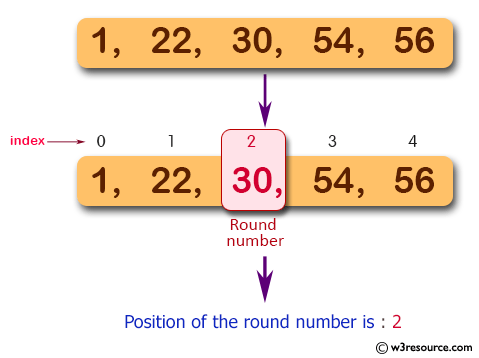# JavaScript: Find the position of a rightmost round number in an array of integers. Returns 0 if there are no round number

## JavaScript Basic: Exercise-139 with Solution

Write a JavaScript program to find the position of a rightmost round number in an array of integers. Returns 0 if there are no round number.

Note: A round number is informally considered to be an integer that ends with one or more zeros.

Pictorial Presentation:Sample Solution:

HTML Code:

``````<!DOCTYPE html>
<html>
<meta charset="utf-8">
<meta name="viewport" content="width=device-width">
<title>Find the position of a rightmost round number in an array of integers. Returns 0 if there are no round number</title>
<body>

</body>
</html>
```
```

JavaScript Code:

``````function find_rightmost_round_number(input_arr) {

var result = 0;
for (var i = 0; i < input_arr.length; i++)
{
if (input_arr[i] % 10 === 0) {
result = i;
}
}

return result;
}

console.log(find_rightmost_round_number([1, 22, 30, 54, 56]));
console.log(find_rightmost_round_number([1, 22, 32, 54, 56]));
console.log(find_rightmost_round_number([1, 22, 32, 54, 50]));
``````

Sample Output:

```2
0
4
```

Flowchart:ES6 Version:

``````function find_rightmost_round_number(input_arr) {

let result = 0;
for (let i = 0; i < input_arr.length; i++)
{
if (input_arr[i] % 10 === 0) {
result = i;
}
}

return result;
}

console.log(find_rightmost_round_number([1, 22, 30, 54, 56]));
console.log(find_rightmost_round_number([1, 22, 32, 54, 56]));
console.log(find_rightmost_round_number([1, 22, 32, 54, 50]));
``````

Live Demo:

See the Pen javascript-basic-exercise-139 by w3resource (@w3resource) on CodePen.

Improve this sample solution and post your code through Disqus

What is the difficulty level of this exercise?

Test your Programming skills with w3resource's quiz.

﻿

## JavaScript: Tips of the Day

Checks if a string is an anagram of another string (case-insensitive, ignores spaces, punctuation and special characters)

Example:

```const isAnagram = (str1, str2) => {
const normalize = str =>
str
.toLowerCase()
.replace(/[^a-z0-9]/gi, '')
.split('')
.sort()
.join('');
return normalize(str1) === normalize(str2);
};
console.log(isAnagram('iceman', 'cinema')); // true
```

Output:

```true
```